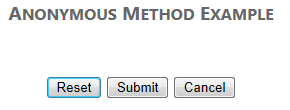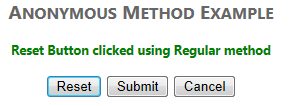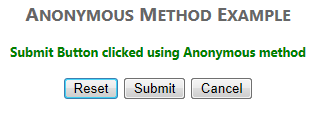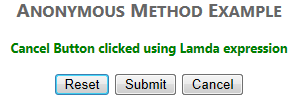# C Sharp Lambda Expression

The concept of lambda expression was introduced in C# 3.0. Basically, Lambda expression is a more concise syntax of anonymous method. It is just a new way to write anonymous methods. At compile time all the lambda expressions are converted into anonymous methods according to lambda expression conversion rules.The left side of the lambda operator "=>" represents the arguments to the method and the right side is the method body.

### Lambda expression Syntax

` (parameters) => expression-or-statement-block `

1. ## Statement Lambda

Statement lambda has a statement block on the right side of the lambda operator "=>".

` x => { return x * x; }; `
2. ## Expression Lambda

Expression lambda has only an expression (no return statement or curly braces), on the right side of the lambda operator "=>".

3. `x => x * x; // here x*x is expression `

## Features of lambda expression

1. Lambda expressions themselves do not have type. In fact, there is no concept of a lambda expression in the CLR.

``` // ERROR: Operator '.' cannot be applied to
// operand of type 'lambda expression'
Type type = ((int x) => x).ToString(); ```
2. A lambda expression cannot be assigned to an implicitly typed local variable since the lambda expressions do not have type.

``` // ERROR: Cannot assign lambda expression to an
// implicitly typed local variable
var thing = (x => x); ```
3. Jump statements (break, goto, continue) are not allowed within anonymous method/lambda expression. Similarly, you cannot jump into the lambda expression/ anonymous method from outside.

4. Variables defined within a lambda expression are accessible only within the scope of the lambda expression body.

5. Lambda expressions are used generally with the Func and Action delegates. our earlier expression can be written as follows:

` Func sqr = x => x * x; `

## Anonymous method with Lambda and delegate

```class Program
{
//delegate for representing anonymous method
delegate int del(int x, int y);

static void Main(string[] args)
{
//anonymous method using expression lambda
del d1 = (x, y) => x * y;
// or (int x, int y) => x * y;

//anonymous method using statement lambda
del d2 = (x, y) => { return x * y; };
// or (int x, int y) => { return x * y; };

//anonymous method using delegate keyword
del d3 = delegate(int x, int y) { return x * y; };

int z1 = d1(2, 3);
int z2 = d2(3, 3);
int z3 = d3(4, 3);

Console.WriteLine(z1);
Console.WriteLine(z2);
Console.WriteLine(z3);
}
}
//output:
6
9
12
```

## Lambda expression as an Event Handler

``` <form id="form1" runat="server">
<div align="center">
<h2>Anonymous Method Example</h2>
<br />
<asp:Label ID="lblmsg" runat="server" ForeColor="Green" Font-Bold="true"></asp:Label>
<br /><br />
<asp:Button ID="btnReset" runat="server" Text="Reset" />
<asp:Button ID="btnSubmit" runat="server" Text="Submit" />
<asp:Button ID="btnCancel" runat="server" Text="Cancel" />
</div>
</form> `````` protected void Page_Load(object sender, EventArgs e)
{
// Click Event handler using Regular method
btnReset.Click += ClickEvent;
// Click Event handler using Anonymous method
btnSubmit.Click += delegate { lblmsg.Text="Submit Button clicked using Anonymous method"; };
// Click Event handler using Lamda expression
btnCancel.Click += (senderobj, eventobj) => { lblmsg.Text = "Cancel Button clicked using Lambda expression"; };
}
protected void ClickEvent(object sender, EventArgs e)
{
lblmsg.Text="Reset Button clicked using Regular method";
} ```##### Summary